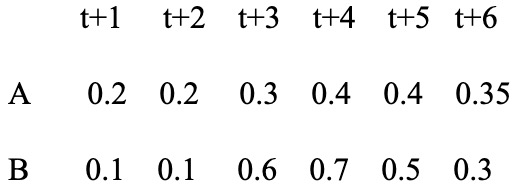### Create an Account

Home / Questions / Show the relevant cash flow present value equation for each answer

# Show the relevant cash flow present value equation for each answer

Show the relevant cash flow/ present value equation for each answer. You must provide one or two brief sentences explaining your result.  For each of the 3 parts, display the appropriate cash flow/ present value equation and give the one or two-line explanation of the logic of your results.

Investors are evaluating two 6-year bonds at time t in an emerging financial crisis setting where there is an increasing likelihood of default. Assume the following values over time for the probability of default (z) of the two bonds, issued respectively by companies A and B.a. Assume both bonds are 6-year, 6% coupon, \$1000 face value coupon bonds, each selling for \$1000. Calculate the yields on the two bonds. Which is higher?

b. Now assume a setting where all future interest rates are exogenously fixed at 5%; the prices of the bond are now to be determined. Determine the prices of bonds A and B? Which is higher?

c. With respect to (b) above, and taking both present value streams together, does there exist some value for i that would make the price of Bond A equal to the price of Bond B?

A B t+1 t+2 t+3 t+4 t+5 t+6 0.2 0.2 0.3 0.4 0.4 0.35 0.1 0.1 0.6 0.7 0.5 0.3

Jan 31 2020 View more View LessGet Solution